Next: Exercises Up: Wave Optics Previous: Multi-Slit Diffraction

# Two-Dimensional Fourier Optics

Consider a monochromatic plane light wave, propagating in the-direction, which is normally incident on an opaque screen that occupies the plane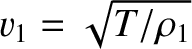. Suppose that there is an irregularly shaped aperture cut in the screen, and that the light that passes through this aperture travels to a flat projection screen occupying the plane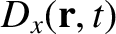. We wish to determine the interference/diffraction pattern that appears on the projection screen, as a function of the size and shape of the aperture. We can achieve this goal by employing a modified version of Huygen's principle. In Section 11.6, we divided the aperture into infinitesimal parallel strips of equal height, and argued that each strip emits a cylindrical wave in the forward direction that has the same amplitude and phase as the light that illuminates it from behind. For the case of an irregularly shaped aperture, we can similarly divide the aperture into infinitesimal squares of equal area, and argue that each square emits a spherical wave in the forward direction that has the same amplitude and phase as the light that illuminates it from behind. [Recall that line sources and point sources emit cylindrical and spherical waves, respectively. (See Sections 8.3 and 8.4.)] Using analogous arguments to those employed in Section 11.2, we can show that provided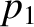(1062)

where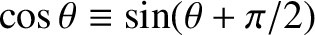is the characteristic size of the aperture, and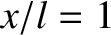is the wavelength of the light that illuminates from behind, we are in the far-field limit. In this limit, the interference/diffraction pattern appearing on the projection screen is entirely due to the phase differences between the spherical waves that travel to a given point on the screen from different parts of the aperture. These phase differences are produced by the slightly different distances traveled by these waves.

Consider a spherical wave emitted from the point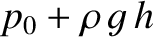,,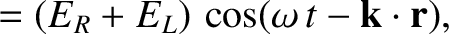in the aperture that travels to the point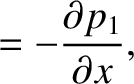,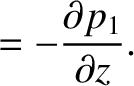,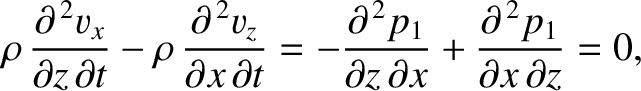on the projection screen. The distance traveled by this wave is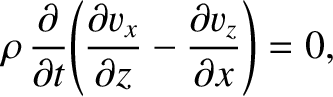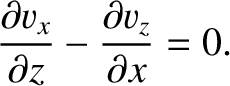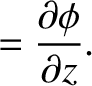(1063)

where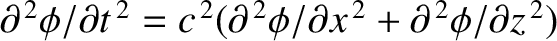and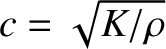are the angular coordinates of the point on the projection screen. Here, we are assuming that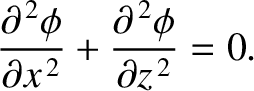(1064)

The wavefunction at the point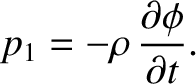,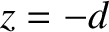on the projection screen is a linear superposition of the spherical waves that travel to it from all parts of the aperture. It follows that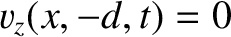(1065)

Here,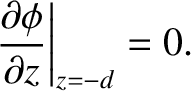is an aperture function that takes the value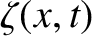in the unblocked parts of the opaque screen, whereis the aperture area, and the value zero in the blocked parts. We have neglected the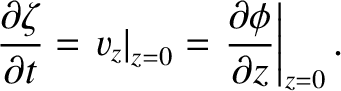variation of the wave amplitudes (see Section 8.4) because we are working in the far-field limit. It follows, from Equations (1063) and (1065), that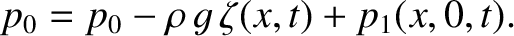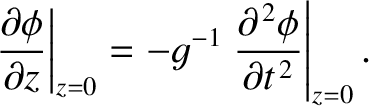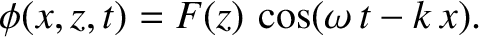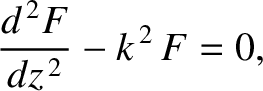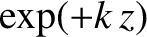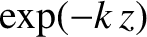(1066)

Here, we have made use of the fact that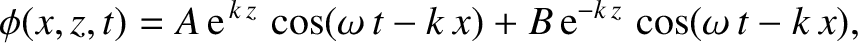,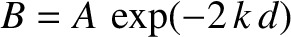, and have also assumed that the aperture function is an even function of both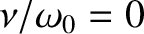and(i.e., the aperture is symmetric about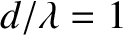and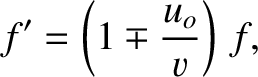). The intensity of the light at the point,on the projection screen is thus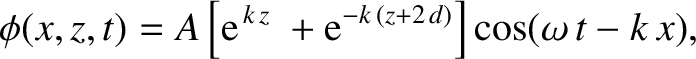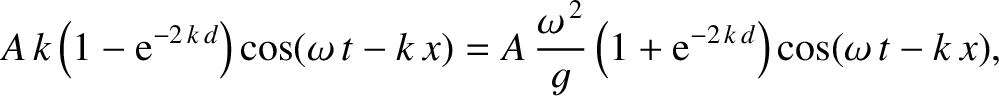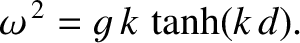(1067)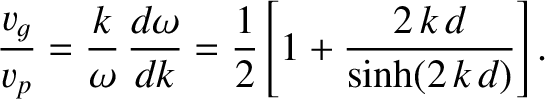Consider a rectangular aperture that occupies the region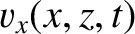and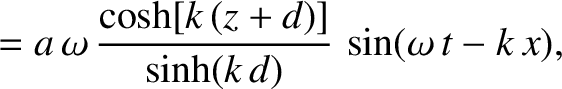in the-plane. The intensity of the interference/diffraction pattern on the projection screen produced by such an aperture is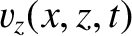(1068)

which yields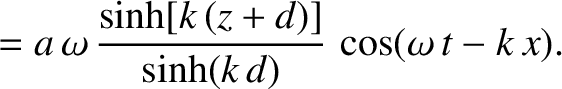(1069)

This pattern is shown in Figure 74. It consists of a strong central maximum, that extends over the region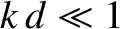and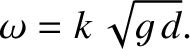, surrounded by much weaker secondary maxima arranged on a rectangular grid. Incidentally, our assumption that,is only self-consistent provided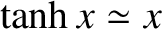,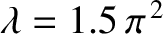(i.e., provided the wavelength of the light is much less than the dimensions of the aperture).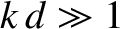Consider a circular aperture that occupies the region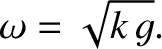in the-plane. Let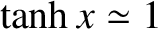,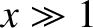,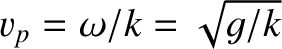, and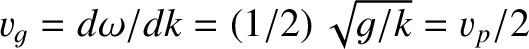. The intensity of the interference/diffraction pattern on the projection screen produced by such an aperture is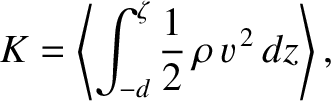(1070)

or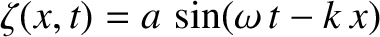(1071)

where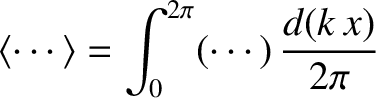. It is readily demonstrated that (Abramowitz and Stegun 1965)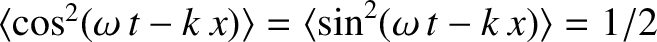(1072)

whereis the Bessel function of degree zero introduced in Section 8.5. It follows that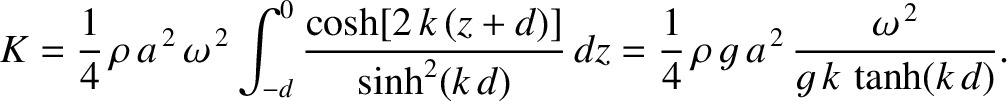(1073)

It can be shown that (Gradshteyn and Ryzhik 1980)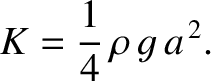(1074)

where (Abramowitz and Stegun 1965)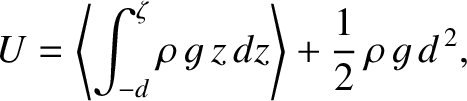(1075)

is a Bessel function of degree one. Hence,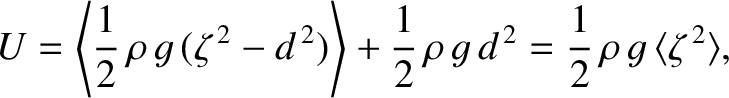(1076)

Figure 75 shows the function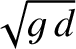, whereas Table 4 lists the first few values ofat which this function is zero. Finally, Figure 76 shows the interference/diffraction pattern associated with a circular aperture. The pattern consists of a central disk, known as an Airy disk, surrounded by much fainter concentric rings. The angular radius of the Airy disk is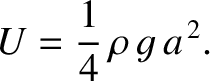where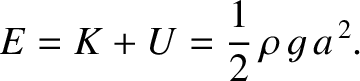is the first zero of the function. It follows that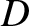(1077)

whereis the diameter of the aperture.

Table: First few zeros of the function. Source: Abramowitz and Stegun 1965.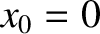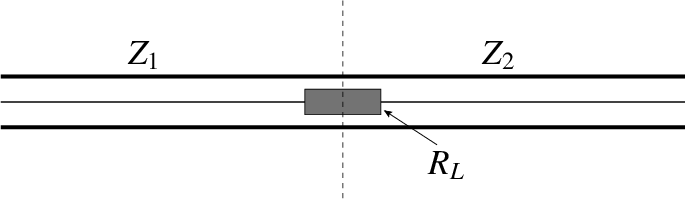1 3.83171 6 19.61586 2 7.01559 7 22.76008 3 10.17347 8 25.90367 4 13.32369 9 29.04683 5 16.47063 10 32.18968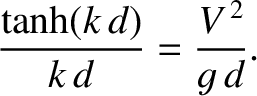As we have already mentioned (see Section 11.7), when a point light source, such as a star, is observed in a telescope, what is actually seen is the diffraction patten of the source produced by the objective aperture. If the aperture is circular then then the telescope images the star as an Airy disk, surrounded by much fainter rings. Likewise, the telescope images two neighboring stars as two Airy disks. The Rayleigh criterion (see Section 11.7) for resolving the two stars (i.e., for being able to tell that there are two stars, rather than one) is that the angular distance,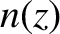, between the stars be less than the angular radius of their Airy disks. This yields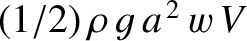(1078)

where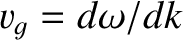is the diameter of the telescope's objective aperture. Thus, the maximum angular resolution of the telescope is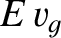(1079)

which is a slightly more accurate version of the criterion given in Section 11.7.Next: Exercises Up: Wave Optics Previous: Multi-Slit Diffraction
Richard Fitzpatrick 2013-04-08